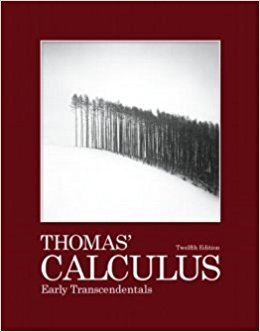# Solutions for Chapter 4: Applications of Derivatives## Full solutions for Thomas' Calculus Early Transcendentals | 12th Edition

ISBN: 9780321588760Solutions for Chapter 4: Applications of Derivatives

Solutions for Chapter 4
4 5 0 256 Reviews
19
5
##### ISBN: 9780321588760

This expansive textbook survival guide covers the following chapters and their solutions. Chapter 4: Applications of Derivatives includes 136 full step-by-step solutions. This textbook survival guide was created for the textbook: Thomas' Calculus Early Transcendentals, edition: 12. Since 136 problems in chapter 4: Applications of Derivatives have been answered, more than 4117 students have viewed full step-by-step solutions from this chapter. Thomas' Calculus Early Transcendentals was written by and is associated to the ISBN: 9780321588760.

Key Calculus Terms and definitions covered in this textbook
• Cosine

The function y = cos x

• Degree

Unit of measurement (represented by the symbol ) for angles or arcs, equal to 1/360 of a complete revolution

• Determinant

A number that is associated with a square matrix

• End behavior

The behavior of a graph of a function as.

• Explanatory variable

A variable that affects a response variable.

• Factored form

The left side of u(v + w) = uv + uw.

• Fitting a line or curve to data

Finding a line or curve that comes close to passing through all the points in a scatter plot.

• Frequency table (in statistics)

A table showing frequencies.

• Linear regression

A procedure for finding the straight line that is the best fit for the data

• Opposite

See Additive inverse of a real number and Additive inverse of a complex number.

• Principal nth root

If bn = a, then b is an nth root of a. If bn = a and a and b have the same sign, b is the principal nth root of a (see Radical), p. 508.

• Quotient identities

tan ?= sin ?cos ?and cot ?= cos ? sin ?

• Sample survey

A process for gathering data from a subset of a population, usually through direct questioning.

• Sequence

See Finite sequence, Infinite sequence.

• Transitive property

If a = b and b = c , then a = c. Similar properties hold for the inequality symbols <, >, ?, ?.

• Variance

The square of the standard deviation.

• Variation

See Power function.

• Vertex of an angle

See Angle.

• Vertical translation

A shift of a graph up or down.

• Yscl

The scale of the tick marks on the y-axis in a viewing window.

×

I don't want to reset my password

Need help? Contact support

Need an Account? Is not associated with an account
We're here to help